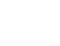Comparing Ratios on the GMATAmong other things, this post is a case study of an OG DS question:

OG 12th Edition, Data Sufficiency Practice Problem #23

Here is the problem that appears in the OG:

23) If p1 and p2 are the populations and r1 and r2 the number of representatives of District 1 and District 2 respectively, the ratio of the population to the number of representatives is greater for which of the following districts?

(1) p1>p2

(2) r2>r1

(A) Statement 1 alone is sufficient but statement 2 alone is not sufficient to answer the question asked.

(B) Statement 2 alone is sufficient but statement 1 alone is not sufficient to answer the question asked.

(C) Both statements 1 and 2 together are sufficient to answer the question but neither statement is sufficient alone.

(D) Each statement alone is sufficient to answer the question.

Below, I’ll show the solution to this.

Basic Fraction Facts

Here are two very important fraction rules.

1) When the numerator increases and the denominator stays the same, the fraction as a whole increases.  When the numerator decreases and the denominator stays the same, the fraction as a whole decreases.

2) When the denominator increases and the numerator stays the same, the fraction as a whole decreases.  When the denominator decreases and the numerator stays the same, the fraction as a whole increases.

Those, as they are stated, are always true 100% of the time.

Not As Simple

If both the numerator and denominator change at the same time, then things are trickier.  See the table below, which assumes that both numerator and denominator are positive.

 Numerator Denominator Fraction as a Whole increases increases (all bets are off) increases decreases increases decreases increases decreases decreases decreases (all bets are off)

In the first case, for example, whether the fraction increases depends on how much the numerator increases, and how much the denominator increases.  For example:

1/3 -> 2/5   both numerator & denominator increase, and fraction increases

1/3 -> 2/7   both numerator & denominator increase, and fraction decreases

(Both of those can be easily seen from the fact that 1/3 = 2/6, so be increasing or decreasing the denominator of 2/6, we can create bigger or smaller fractions.)

Back to the OG DS Problems

Now, we can tackle the DS problem above.  We are comparing fractions of population over number of representative, p/r.

Statement #1: The numerator of the first fraction is larger.  If we knew the denominators were staying the same or getting smaller, we could deduce that the fraction overall is getting bigger.  Unfortunately, we have no information about how the denominators compare.  Statement #1, by itself is insufficient.

Statement #2: As always in GMAT DS question, we have to forget about everything in Statement #1 and focus only on what Statement #2 says.  — We know the second fraction has a lager denominator.  We have no information now about the numerators.  Statement #2, by itself is insufficient.

Statements #1 & #2 combined: Now, we know that when we go from the first fraction to the second fraction,the numerator decreases and the denominator increases.  This is row three of the table above: the fraction as a whole decrease, so District #2 has a lower population to representative ration than District 1.  Answer = C

Here’s another GMAT Data Sufficiency Question on ratios, to practice: http://gmat.magoosh.com/questions/995

Author

•Mike served as a GMAT Expert at Magoosh, helping create hundreds of lesson videos and practice questions to help guide GMAT students to success. He was also featured as "member of the month" for over two years at GMAT Club. Mike holds an A.B. in Physics (graduating magna cum laude) and an M.T.S. in Religions of the World, both from Harvard. Beyond standardized testing, Mike has over 20 years of both private and public high school teaching experience specializing in math and physics. In his free time, Mike likes smashing foosballs into orbit, and despite having no obvious cranial deficiency, he insists on rooting for the NY Mets. Learn more about the GMAT through Mike's Youtube video explanations and resources like What is a Good GMAT Score? and the GMAT Diagnostic Test.